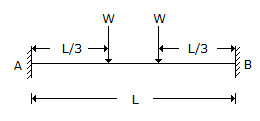# Civil Engineering - UPSC Civil Service Exam Questions

21.

Consider the following statements :
1. Welded structures are usually lighter than riveted structures.
2. Welding allows the arrangement of structural components in such a manner that the joint provides maximum efficiency.
3. During welding, the members do not get distorted.
Of these statements :

 A. 1, 2 and 3 correct B. 1 and 2 are correct C. 2 and 3 are correct D. 1 and 3 are correct

Explanation:

No answer description available for this question. Let us discuss.

22.

Which is the major pollutant present in photochemical smog ?

 A. PAN B. S02 C. HC D. N02

Explanation:

No answer description available for this question. Let us discuss.

23.

What are the bending moments at the ends A and B of a uniform beam AB fixed in direction and position at A and B when acted upon by two concentrated loads at l/3rd span as shown in the figure ?A. 2/9 WL B. 4/9 WL C. 6/9 WL D. 8/9 WL

Explanation:

No answer description available for this question. Let us discuss.

24.

Due to rise in temperature, the viscosity and unit weight of percolating fluid are reduced to 70% and 90% respectively. Other things being constant, the change in coefficient of permeability will be

 A. 20.0% B. 28.6% C. 63.0% D. 77.8%

Explanation:

No answer description available for this question. Let us discuss.

25.

The correct sequence of the sludge digestion steps is

 A. Acid formation, hydrolysis, methane formation B. Methane formation, acid formation, hydrolysis C. Hydrolysis, methane formation, acid formation D. Hydrolysis, acid formation, methane formation